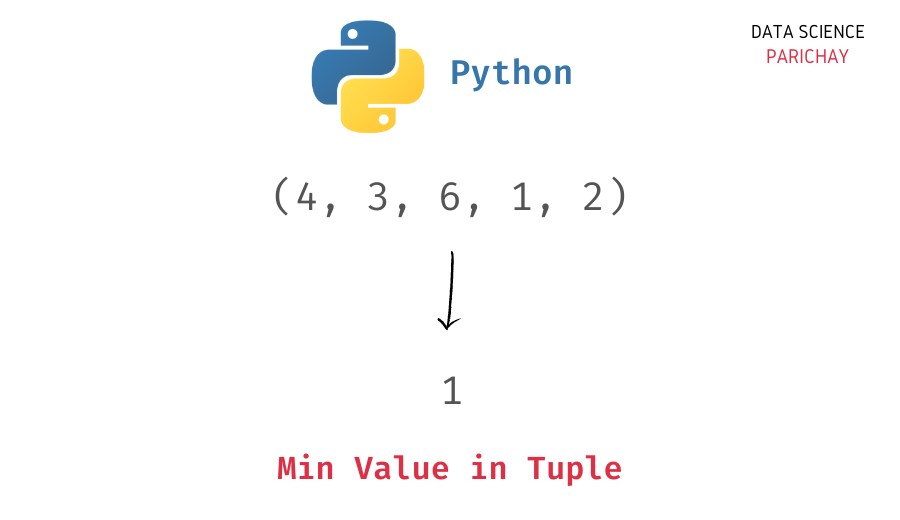# Get Min Value and its Index in a Tuple in Python

In this tutorial, we will look at how to get the min value and its corresponding index in a tuple in Python with the help of some examples.

You can use the Python built-in `min()` function to get the min value in a tuple. Alternatively, you can also iterate through the tuple to find the minimum value.

Let’s look at the two methods with the help of examples.

The Python built-in `min()` function returns the minimum value in an iterable. Pass the tuple as an argument to get its minimum value.

```# create a tuple
t = (2, 7, 5, 1, 4)
# get min value in tuple
print(min(t))```

Output:

`1`

We get the minimum value of the tuple `t` as `1`.

To get the index corresponding to the min value, you can use the tuple’s `index()` function. This function returns the index of the first occurrence of the element inside the tuple. If the element is not present, it raises an error.

```# create a tuple
t = (2, 7, 5, 1, 4)

# find min value
min_val = min(t)
# display its index
print(t.index(min_val))```

Output:

📚 Data Science Programs By Skill Level

Introductory

Intermediate ⭐⭐⭐

🔎 Find Data Science Programs 👨‍💻 111,889 already enrolled

Disclaimer: Data Science Parichay is reader supported. When you purchase a course through a link on this site, we may earn a small commission at no additional cost to you. Earned commissions help support this website and its team of writers.

`3`

We get the index of the min value as 3.

Alternatively, you can iterate through each value in the tuple and keep track of the minimum value to find the min value in the tuple. You can use an additional variable to keep track of the index of the minimum value.

Let’s look at an example.

```# create a tuple
t = (2, 7, 5, 1, 4)

# find min value using loop
min_val = t
min_val_idx = 0
for i in range(len(t)):
if t[i] < min_val:
min_val = t[i]
min_val_idx = i

# display the min value
print(min_val)
# display its index
print(min_val_idx)```

Output:

```1
3```

We get the minimum value and its index in the tuple.

Note that if your tuple contains more than one occurrence of a value, the `index()` function will only return its first occurrence. To get all the indexes of occurrence, you iterate through the elements in a list comprehension.

```# create a tuple
t = (1, 7, 5, 1, 7)

# get all indexes of 1
idx = [i for i in range(len(t)) if t[i] == 1]
print(idx)```

Output:

`[0, 3]`

We get all the indexes of occurrence for the value `1` in the tuple `t`.

You might also be interested in –

•